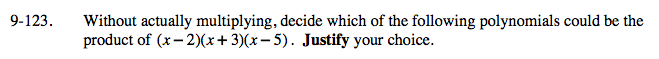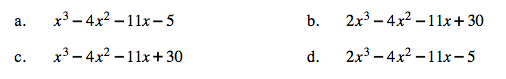Home > A2C > Chapter 9 > Lesson 9.3.1 > Problem9-123

9-123.
1. Without actually multiplying, decide which of the following polynomials could be the product of (x − 2)(x + 3)(x − 5). Justify your choice. Homework Help ✎

1. x3 − 4x2 − 11x − 5

2. 2x3 − 4x2 − 11x + 30

3. x3 − 4x2 − 11x + 30

4. 2x3 − 4x2 − 11x − 5Polynomial (c) because (−2)(3)(−5) = 30 and (x)(x)(x) = x3 not 2x3.